# Use formulas

Formulas let you calculate the value of a topic property by evaluating an expression that uses other topic properties from specific topics or a range of topics on your map. The properties used in the calculation are called input properties, and the resulting property is called a calculated property. Any topic on your map can contain one or more formulas to define one or more calculated properties.

Calculated properties are updated dynamically, so their values are automatically updated as you add or edit the input properties, or move, add or remove their topics.

Formulas can use input properties whose type is number, currency, integer, percentage or calculated.

• If you used AutoCalc in an earlier version of MindManager, a formula is created for each calculated property you defined. If you edit these formulas in MindManager 14, they will not be supported in earlier versions of MindManager.

### What is a formula?

Example of a simple formulas are:

[unit_cost] = 22 + 3

Meaning: the "unit_cost" topic property is equal to 22 plus 3.

[unit_price] = [unit_cost] * 1.25

Meaning: the "unit_price" topic property for this topic equals the "unit_cost" property times 1.25.

[product_profit] =  ([unit_price] - [unit_cost]) *[units]

Meaning: the "product_profit" topic property equals the "unit_price" property minus the "unit_cost" property times the number of units.

You can use topics anywhere on the map in the calculation, by using a formula like this:

[total_profit] = SUM ( MapTopics [product_profit])

Meaning: the "total_profit" topic property for this topic equals the sum of the "product_profit" properties of all topics in all map topics.

You can also create more complex calculations that can evaluate a range of topics anywhere on the map and include only specific topics or topic types.

An example of a more sophisticated formula that you can create in the Formula Editor is:

[total_budget_surplus] = SUM(MapTopics [budget]) - SUM (MapTopics[travel_expense],MapTopics[equipment_expense],MapTopics[salaries],topic[operations_expense])

Meaning: the "total_budget_surplus" topic property for this topic equals the sum of the "budget" property on all map topics, minus the sum of the "travel_expense", "equipment_expense", and "salaries" properties on all map topics, and the "operations_expense" property on a specific map topic.

### How do I create formulas?

Use the buttons on the Advanced tab to enter formulas: AutoCalc provides an interface for easy formula creation, or click Formulas to use the Formula Editor to create or edit more complex formulas.

• The choice of decimal separators (period or comma) and list separators (comma or semicolon) is determined by the Windows Control Panel Region and Language settings for your computer.

What do you want to do?

## Create a simple formula with AutoCalc

Autocalc lets you create simple formulas that include a single function, one input property, and one range.

To create more sophisticated formulas, use the Formula Editor:

1. Select a topic on the map.

2. On the ribbon's Advanced tab, click AutoCalc.

1. In the dialog, select:

• The calculated property to be created on this topic by the formula.

• The Function to use in the calculation.

• The input property used by the function.

• The topic range to evaluate.

1. Select the checkbox to add the input property to topics that are used in the calculation but do not already contain this property. Select the property type for these properties.
(Use this option if you expect all the topics used in the calculation to contain the input property - you can fill in the input property values at any time. If the input property is not present on a topic, that topic is ignored by the calculation)

2. Click OK.

Once you have created the formula, the topic will display a formula icon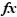. You can see a list of the topic's formulas by pointing to this icon.

If you want to change the formula you created, you can edit it in the Formula Editor.

• You can see all the topics that contain formulas on the Map Index Elements tab.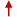Top of Page

## Create a formula with the Formula Editor

The Formula Editor lets you create sophisticated formulas that include multiple functions (with grouping for precedence), input properties, and ranges.

1. Select a topic on the map.

2. On the ribbon's Advanced tab click Formulas.

3. The Formula Bar appears, and shows all the formulas contained on the selected topic.

4. Click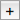to open the Formula Editor and enter the new formula.

1. On the Formula Editor Insert bar, click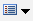to choose the topic property you want to define by the calculation (this is the formula's left-hand side). If you choose Other, enter the name for the new property between the brackets that appear [  ].

2. Click to the right of the = and type the formula. For speedier entry, you can use the Insert bar buttons to choose functions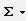, ranges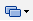, or select specific map topics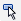to use in the calculation.

See Formula elements and syntax in this topic for details.

3. Click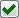to validate the formula. Errors will appear on the Formula Editor status bar.

To cancel changes you made using the Formula Editor, click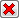.

Once you have created the formula, the topic will display a formula icon.

• You can duplicate the formula on other topics by copying the property that it calculates.

You can see all the topics that contain formulas on the Map Index Elements tab.Top of Page

## Edit or remove a formula

1. Select the topic that contains the formula you want to change or delete.

2. If  the Formula Bar is not displayed, on the ribbon's Advanced tab click Formulas, or click the formula iconon the topic.

3. On the Formula Bar select the formula you want to edit or remove. The Formula Editor starts.

4. To edit the formula, modify the formula text manually or by using the Formula Editor buttons to specify Topic Properties, Functions, Rangesand to select topics.
To remove the formula, click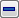.

5. You can also remove a formula from a topic by deleting the topic property that it defines.Top of Page

## Formula elements and syntax

### General form

The general form for a formula is: lhs = rhs

lhs (left-hand side) specifies the calculated topic property to be defined by this formula. It will receive the results of the rhs (right-hand side).

rhs (right-hand side) is an expression that is evaluated to produce a numeric value. This value is assigned to the calculated topic property specified by lhs.
This expression usually acts on a range of topics and / or on specific topics.

### Topic properties

In the Formula Editor, clickto insert a topic property in the equation. On the lhs, you can select only properties in the current topic or create a new property. On the rhs, you can select any property used in the map.

Topic properties are always enclosed in square brackets [  ].
Examples: [cost]  [unit_cost]  [all costs]

Topic properties used in formula calculations must be of one of the following numeric types:

• Number

• Currency

• Percentage

• Integer

• Calculated (a property whose value is determined by another formula)

• You can change the numeric property types (Number, Currency, Percentage, or Integer) to any one of the other three without deleting any data that has already been entered into the property.

### Operators and numbers

 + addition -  (hyphen) subtraction *  (asterisk) multiplication /   (forward slash) division ^ exponentiation ( )  (parentheses) grouping for precedence and enclose function arguments

You can use numbers in equations and group operations with parentheses.

Example:

[Total] = 3*(5+2)+10*(2+2)

[Weighted_Profit] = 3* [Profit] - (2*([Cost] / 15))

### Topic ranges or selection

Topic ranges are used in the formula's right-hand side to specify a set of topics to use in the calculation. You can specify the range by clickingin the Formula Editor, or by typing the keywords below.

To refer to specific topics, clickand then select the topic in the map. If you do not specify a range for a property the calculation uses that property from the current topic. This is displayed in the formula as topic . You can see the name of the map topic by pointing to this text in the Formula Editor, or double-click it to navigate to the topic it references.

• If you use a range that contains topics hidden by a filter, the properties on those topics are ignored during the formula calculation. If you reference a specific topic, its properties are always included in the calculation even if the topic is hidden.

 Self this topic; implicit, if range is not otherwise specified Parent parent of this topic; evaluates to nothing for the Central Topic Branch this topic and all its descendants Children the immediate subtopics (1 level) of this topic Descendants all subtopics (multiple levels) of this topic Siblings all other topics that have the same parent as this topic (not including this topic) Ancestors all topics (multiple levels) from which this topic descends, not including this topic CentralTopic the map's central topic MapTopics all topics in the map, including callouts and floating topics Callouts callouts on this topic; does not include callouts on boundaries and relationships Floating all floating topics in map, not including their descendants

#### Range Examples

[cost] - "The cost property of this topic." (No range is specified, so "self" is implied.)

Siblings [cost] - "The cost property of the siblings of this topic."

topic [cost] - "The cost property of the topic that was selected." (Point to topic  to see the name of the topic referenced.)

### Built-in functions

 SUM The sum of the property values. AVERAGE The sum of the property values divided by the number of properties. COUNT The number of properties. MIN The smallest value of the property within the range. MAX The largest value of the property within the range.

Function arguments are enclosed in parentheses.

Example: [Total_cost] = SUM ( Descendants [cost] )

Multiple arguments are separated by , (commas).

Examples:

[Project_expense] = SUM ([travel_expense],[equipment_expense],[salaries])

[Total_expense] = SUM (MapTopics[Project_expense] , topic[Operations_expense])